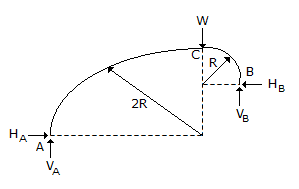# Civil Engineering - UPSC Civil Service Exam Questions

1.

Which one of the following pairs is correctly matched ?

 A. Sandstone....Igneous rock B. Limestone....Sedimentary rock C. Basalt....Metamorphic rock D. Granite....Argiliaceous rock

Explanation:

No answer description available for this question. Let us discuss.

2.

A line of true length 500 m when measured by a 20 m tape is reported to be 502 m long. The correct length of the tape is

 A. 19.92 m B. 20.08 m C. 20.80 m D. 21 m

Explanation:

No answer description available for this question. Let us discuss.

3.

Where should splices in the columns be provided ?

 A. At the floor levels B. At the mid height of columns C. At the beam-column joints D. At one-fourth height of columns

Explanation:

No answer description available for this question. Let us discuss.

4.

In a rectangular channel, the depths of flow before and after the hydraulic jump are respectively 0.2 m and 1.2 m. What is the energy loss in the jump ?

 A. 1.042 m B. 2.084 m C. 3.126 m D. 4.168 m

Explanation:

No answer description available for this question. Let us discuss.

5.

A three-hinged circular arch ACB is formed by two quadrants of circles AC and BC of radii 2R and R respectively with C as crown, as shown in the figure at side. Consider the following in respect of the reactive forces developed at support A and B due to concentrated load W at the Crown.
1. Line of action RA at A is inclined as 45° to the horizontal.
2. VA = VB = W/2
3. HB = 2 HA
4. HA = HB = W/2
Which of the above is/are correct ?A. 1 and 3 B. 2 and 3 C. 1, 2 and 4 D. 4 only